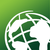Very long computation time for focal medians on a high-resolution DEM

275
2
05-17-2019 04:21 AMNew Contributor

Hello. I need to have focal medians calculated on a DEM, which has floating point values. Its important for me to preserve the tenth/hundredth accuracy. Focal statistics cannot apply non-linear functions like medians on floating point values. Therefore, I am turning the values to integers first (and multiply them by 100 to preserve hundredths). I noticed however, that while calculating MEAN as a kernel function takes several minutes,  MEDIAN can take up to 22 hours in case of my data. I am using a standard/high-end desktop computer with 16 GB ram. Checked on another machine and rasters of similar resolutions and its the same.  My question is; why do medians require so much time to be calculated? Is this the actual reason why the medians cannot be calculated on floats (detrimental computation time)? Thank you for any help.

2 RepliesbyMVP Esteemed Contributor

Even in numpy array representations of rasters using focal calculations (moving/strided windows), the median takes about 4X longer on small arrays

This would scale if the window size is larger since there is more 'sorting' needed to arrange the values for median determination.  Here is an example for a small floating point array with a 3x3 moving window and a median and mean calculation.  If you have nodata values the np.mean and np.median would have to be replaced with np.nanmean and np.nanmedian respectively.  It would take longer still to mask out the nodata values prior to the calculations

import arraytools as art
a = np.random.randint(0, 10, (1000,1000))*1.

arr = art.stride(a)
av = np.mean(arr, axis=(1,2))
md = np.median(arr, axis=(1,2))

%timeit np.mean(arr, axis=(1,2))
34.5 ms ± 263 µs per loop (mean ± std. dev. of 7 runs, 10 loops each)

%timeit np.median(arr, axis=(1,2))
146 ms ± 3.45 ms per loop (mean ± std. dev. of 7 runs, 10 loops each)‍‍‍‍‍‍‍‍‍‍‍‍New Contributor

Dan,

Thanks for checking it this way.

So its probably the matter of a sorting algorithm, which needs to be applied to find median.

Thank you very much,

Jan